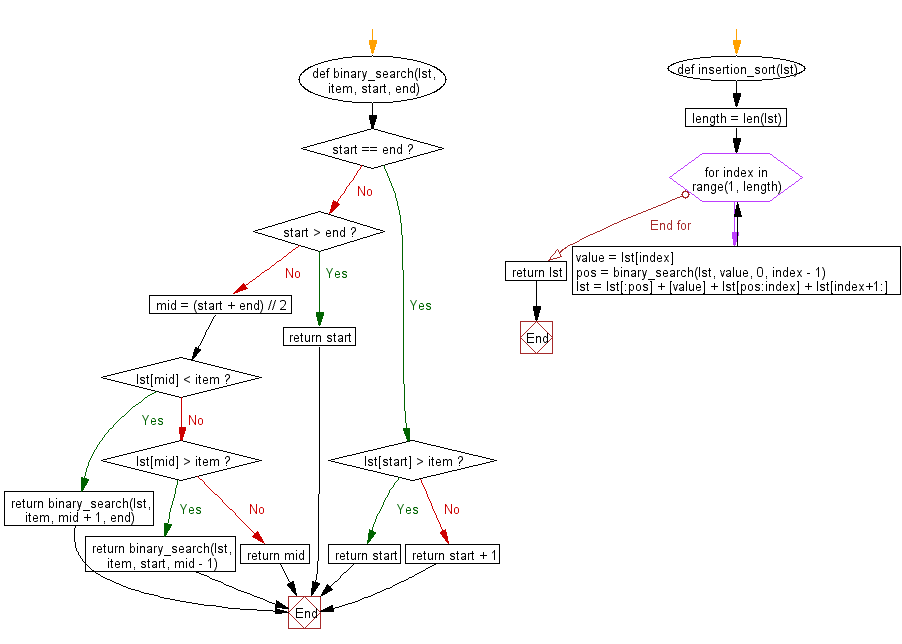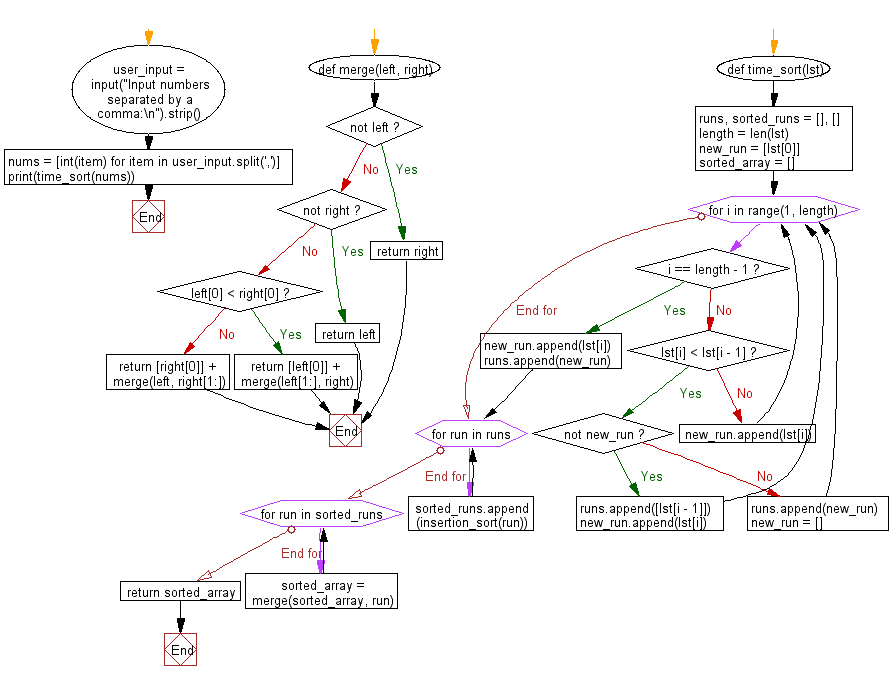﻿ Python: Sort a list of elements using Time sort - w3resource# Python: Sort a list of elements using Time sort

## Python Search and Sorting : Exercise-21 with Solution

Write a Python program to sort a list of elements using Time sort.

Sample Solution:

Python Code:

``````# License https://bit.ly/2InTS3W
def binary_search(lst, item, start, end):
if start == end:
if lst[start] > item:
return start
else:
return start + 1
if start > end:
return start

mid = (start + end) // 2
if lst[mid] < item:
return binary_search(lst, item, mid + 1, end)
elif lst[mid] > item:
return binary_search(lst, item, start, mid - 1)
else:
return mid
def insertion_sort(lst):
length = len(lst)

for index in range(1, length):
value = lst[index]
pos = binary_search(lst, value, 0, index - 1)
lst = lst[:pos] + [value] + lst[pos:index] + lst[index+1:]
return lst

def merge(left, right):
if not left:
return right

if not right:
return left

if left < right:
return [left] + merge(left[1:], right)

return [right] + merge(left, right[1:])

def time_sort(lst):
runs, sorted_runs = [], []
length = len(lst)
new_run = [lst]
sorted_array = []

for i in range(1, length):
if i == length - 1:
new_run.append(lst[i])
runs.append(new_run)
break

if lst[i] < lst[i - 1]:
if not new_run:
runs.append([lst[i - 1]])
new_run.append(lst[i])
else:
runs.append(new_run)
new_run = []
else:
new_run.append(lst[i])

for run in runs:
sorted_runs.append(insertion_sort(run))

for run in sorted_runs:
sorted_array = merge(sorted_array, run)

return sorted_array

user_input = input("Input numbers separated by a comma:\n").strip()
nums = [int(item) for item in user_input.split(',')]
print(time_sort(nums))
```
```

Sample Output:

```Input numbers separated by a comma:
25, 45, 69, 79, 23
[23, 25, 45, 69, 79]
```

Flowchart:What is the difficulty level of this exercise?

Test your Programming skills with w3resource's quiz.

﻿

## Python: Tips of the Day

Use Reversed() In for Loops:

```>>> tasks = ['laundry', 'picking up kids', 'gardening', 'cooking']